Function Repository Resource:

# MirrorManipulate

Generate a graphic of a ray diagram in which the circular mirror parameters can be manipulated dynamically

Contributed by: Julián Laverde
 ResourceFunction["MirrorManipulate"][] returns a manipulable graphic of the ray diagram of a spherical mirror. ResourceFunction["MirrorManipulate"][assoc] returns a manipulable graphic of the ray diagram of a spherical mirror with the corresponding parameters in the Association assoc.

## Details and Options

The available mirrors are convex and concave.
ResourceFunction["MirrorManipulate"] follows the sign convention for spherical mirrors from Hecht, Optics, Pearson, 2015:
 Object distance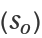(+) real object (-) virtual object Focal length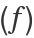(+) converging mirror (-) diverging mirror Image distance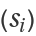(+) real image (-) virtual image Object size (+) erect object (-) inverted object Image size (+) erect image (-) inverted image Magnification (M) (+) erect image (-) inverted image
The linear magnification factor of the system is given by the equation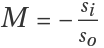, defined as the ratio of the size of the image compared to the size of the object.
ResourceFunction["MirrorManipulate"] uses the Gaussian mirror formula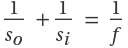to calculate the image distance (si). This equation is only valid for the paraxial region of the mirror.
The arrow in the rays represents the direction of light travel and dotted lines the projection of the rays.
Real images and virtual images are represented by a thick-line arrow and a dotted-line arrow, respectively.
In ResourceFunction["MirrorManipulate"][assoc], the Association assoc can contain the following elements:
 "Diameter" 4 mirror (half) diameter "ObjectSize" 0.5 object initial size "FocalLength" "Diameter" mirror initial focal length "ObjectDistance" "FocalLength" object initial position
"ObjectDistance" can be a positive number or quantity of length.
"ObjectSize" can be a number in the range[-1,1].
"FocalLength" can be a positive or negative number or quantity of length.
Quantity inputs should have units of length. Results and values in sliders will be shown in the most common unit among the inputs.
ResourceFunction["MirrorManipulate"][] takes the options of Graphics and the following:
 "RayColor" Automatic specify the colors of the rays "MirrorColor" Automatic specify the color of the mirror "ArrowColor" Automatic specify the colors of the object and image arrows "ShowEquations" True set the equations in the graphics object "ObjectDistanceRange" Automatic specify the range of "ObjectDistance" "ObjectSizeRange" Automatic specify the range of "ObjectSize" "FocalLengthRange" Automatic specify the range of "FocalLength"
The copy values button copies the current values of "ObjectDistance", "FocalLength", "ImageDistance" and "Magnification" to the clipboard as an Association.

## Examples

### Basic Examples (2)

Launch MirrorManipulate, a Manipulate about mirrors:

 In:=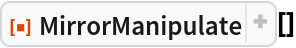Out=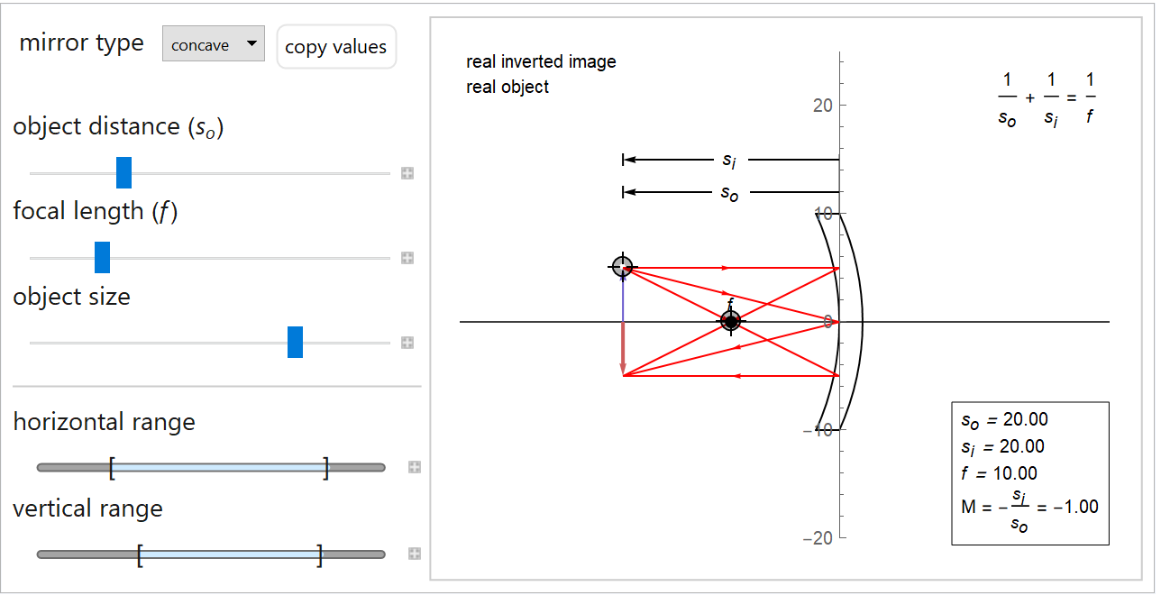Get the ray diagram for a set of specified initial values:

 In:=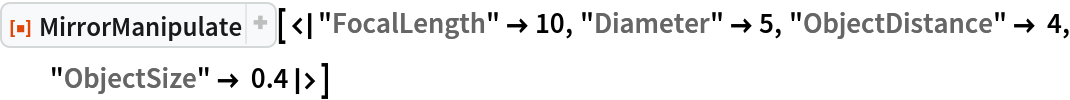Out=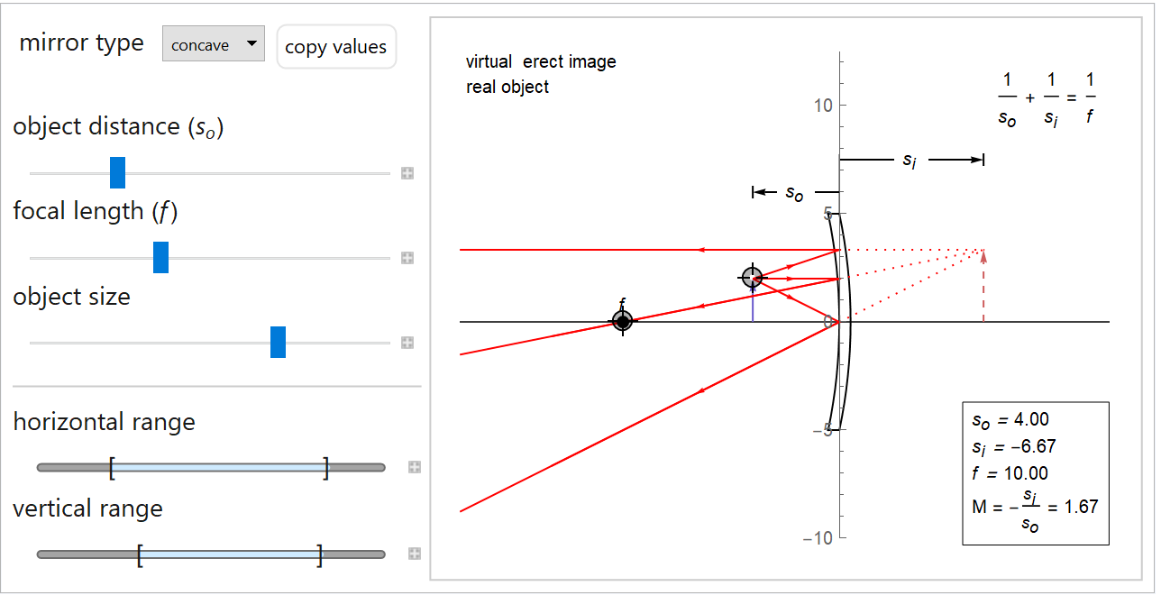### Scope (2)

Get the ray diagram for a negative mirror:

 In:=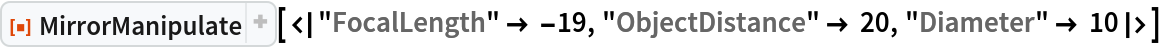Out=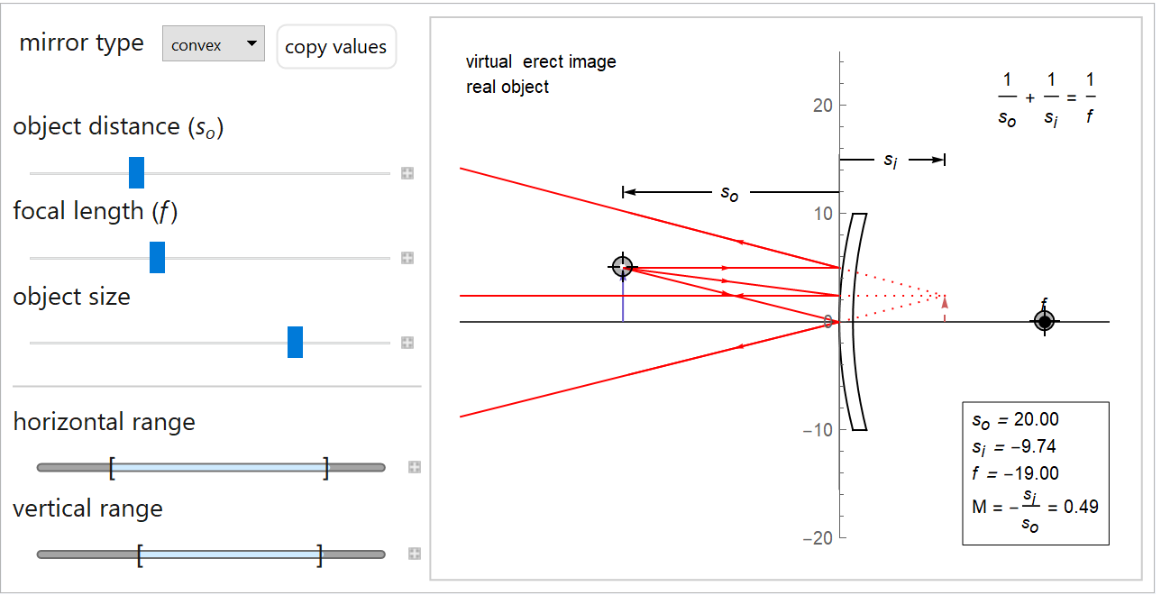Use quantities to specify lengths:

 In:=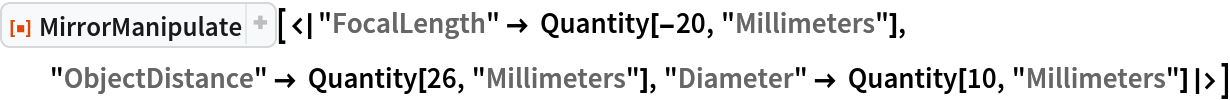Out=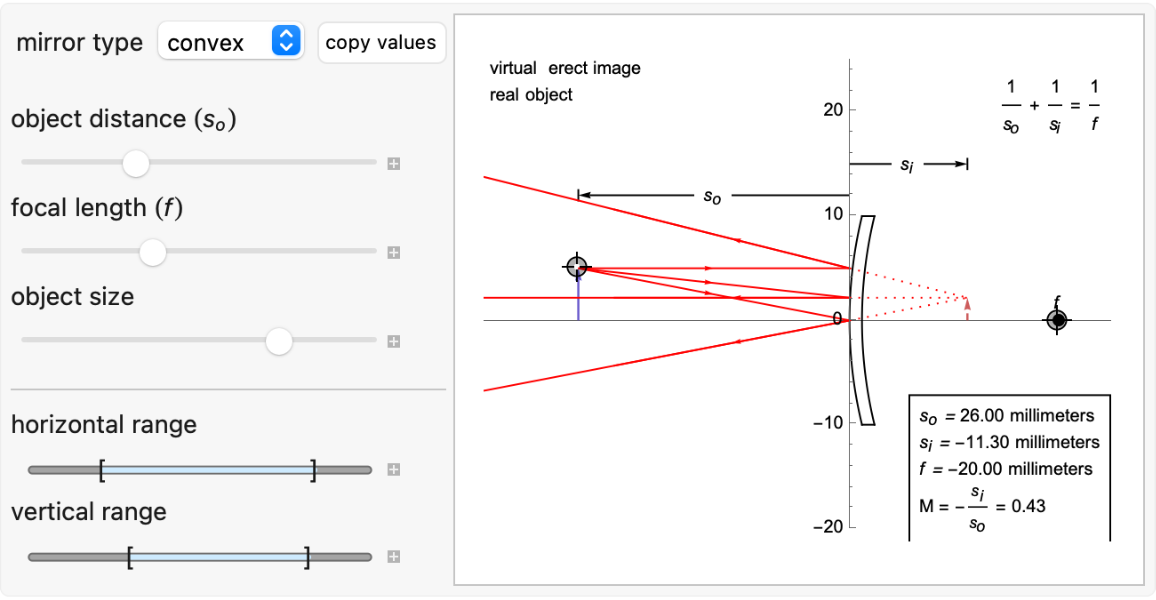### Options (7)

LensManipulate takes the options of Graphics:

 In:=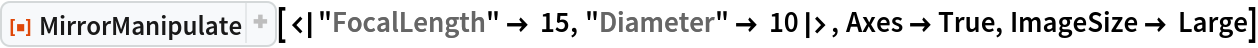Out=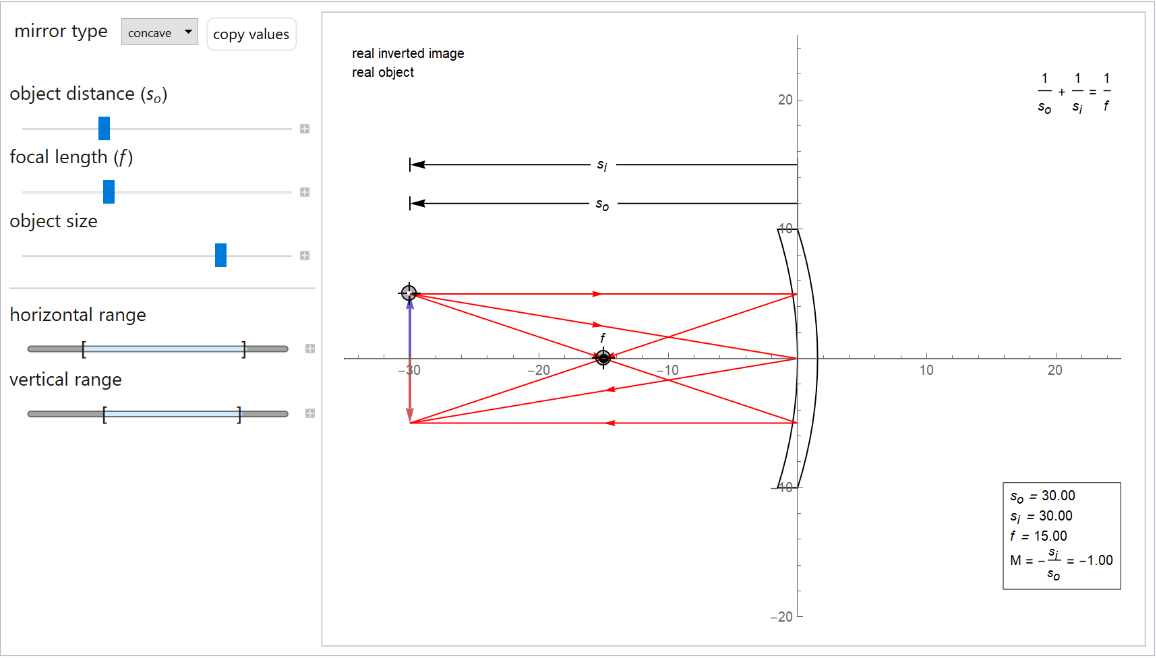#### ShowEquations (1)

Set "ShowEquations" to False:

 In:=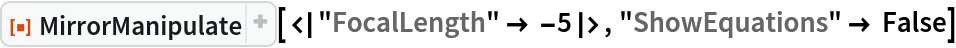Out=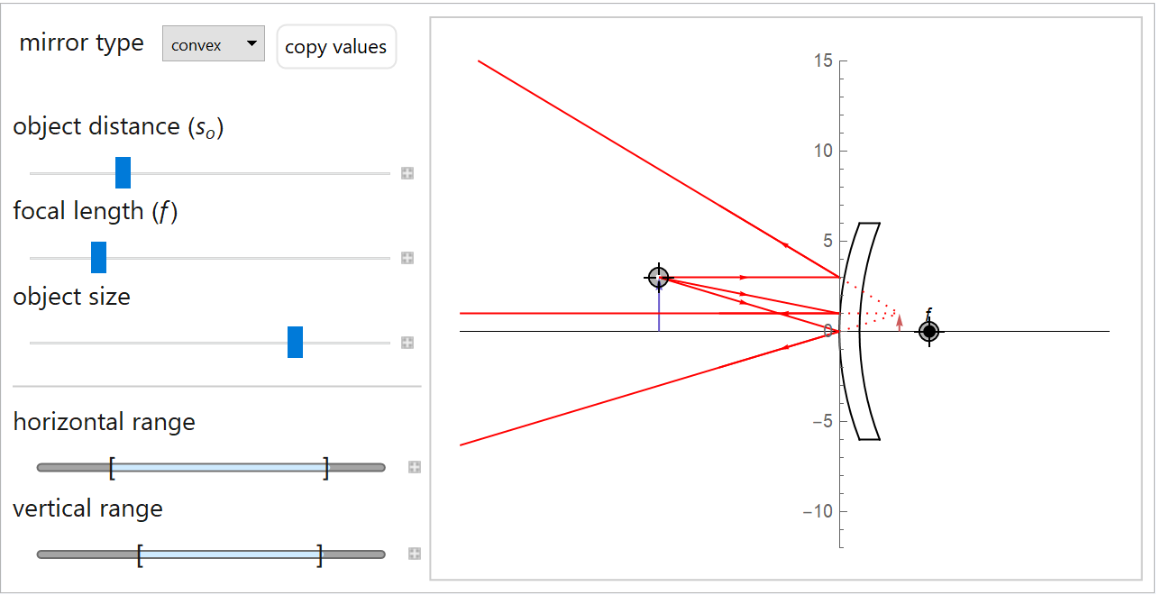#### RayColor (1)

Change the color of the rays:

 In:=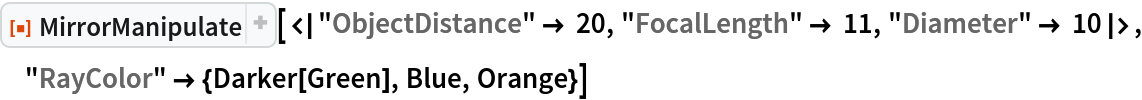Out=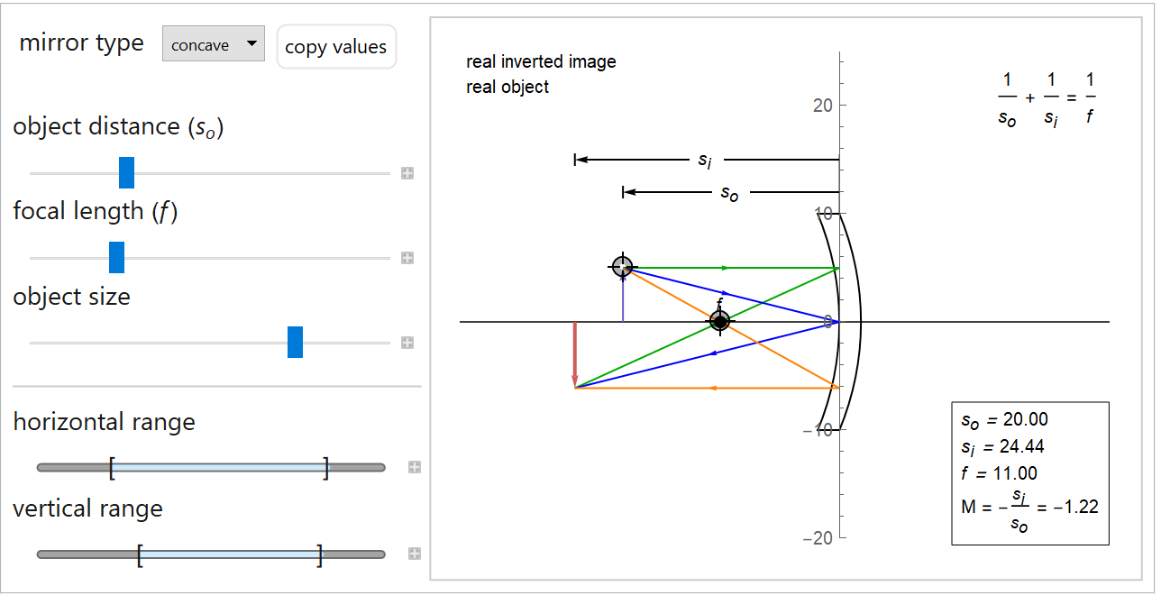#### ArrowColor (1)

Change the color of the object and virtual arrows:

 In:=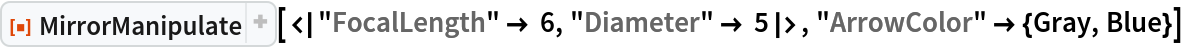Out=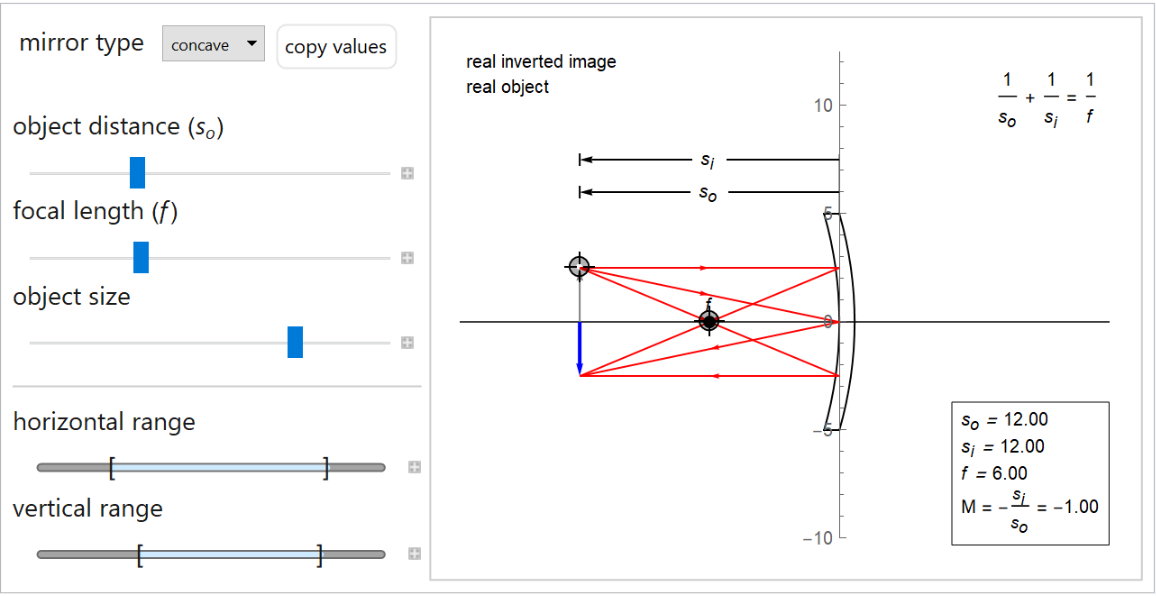#### MirrorColor (1)

Specify the color of the mirror:

 In:=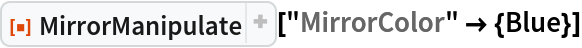Out=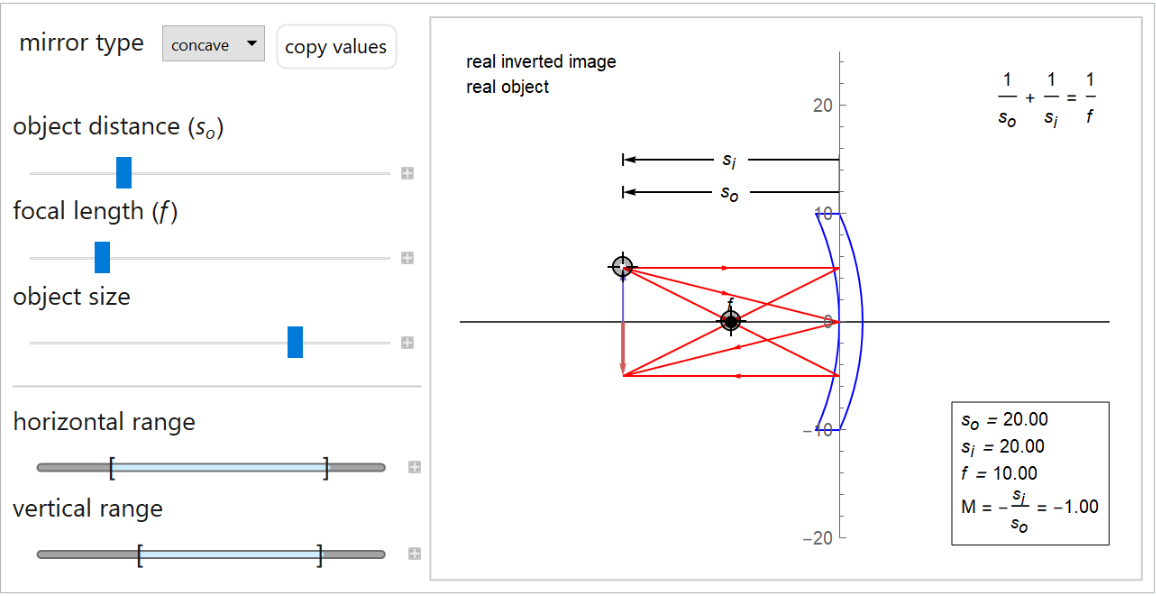#### ObjectDistanceRange (1)

Specify the range of "ObjectDistance":

 In:=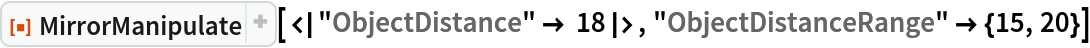Out=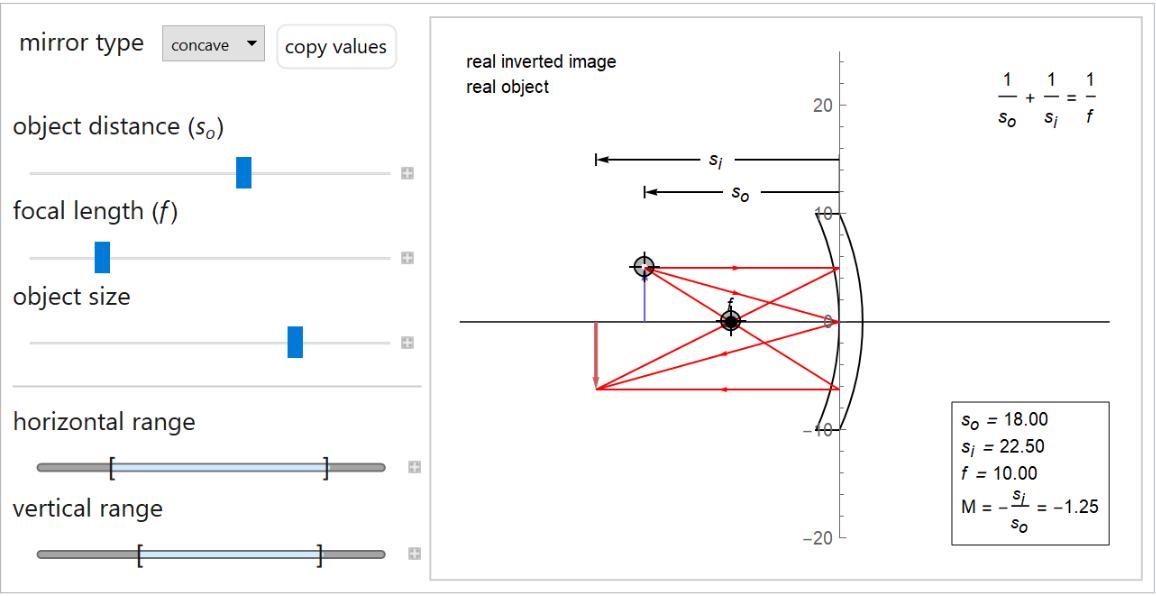#### ObjectSizeRange (1)

Specify the range of "ObjectSize":

 In:=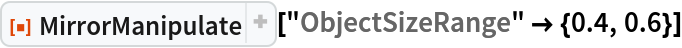Out=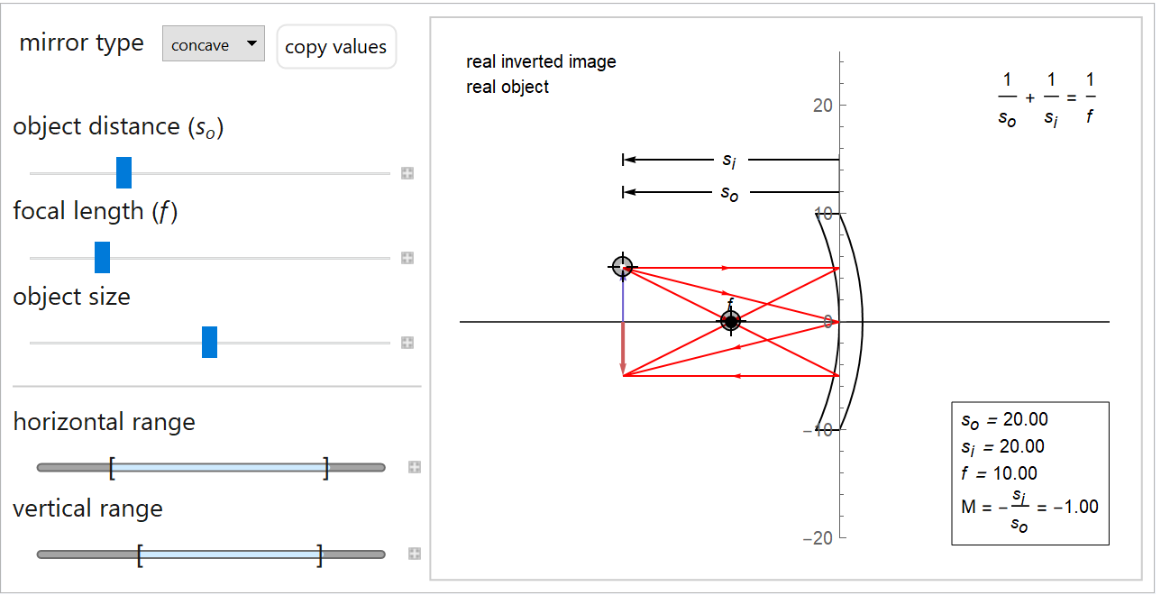#### FocalLengthRange (1)

Specify the range of "FocalLength":

 In:=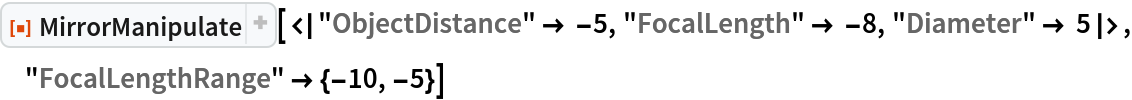Out=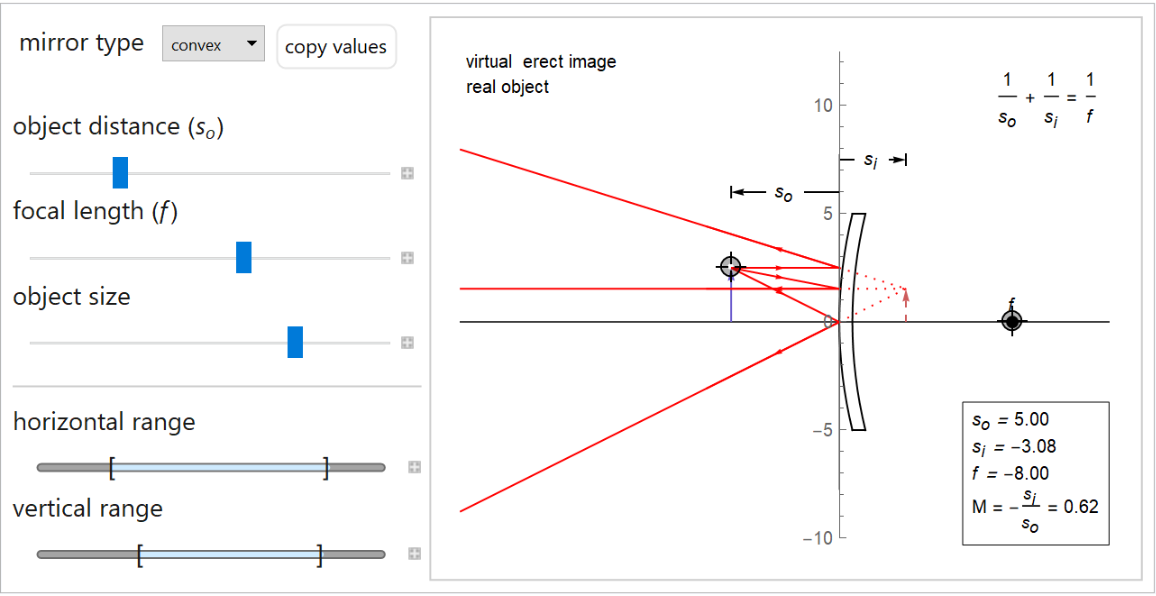## Publisher

WolframSpecialProjects

## Version History

• 1.2.0 – 12 September 2022
• 1.1.0 – 02 June 2021
• 1.0.0 – 17 March 2021